# PSEB 7th Class Maths Solutions Chapter 9 Rational Numbers Ex 9.1

Punjab State Board PSEB 7th Class Maths Book Solutions Chapter 9 Rational Numbers Ex 9.1 Textbook Exercise Questions and Answers.

## PSEB Solutions for Class 7 Maths Chapter 9 Rational Numbers Ex 9.1

1. Write two equivalent rational numbers of the following :

Question (i).
$$\frac {4}{5}$$
Solution:
$$\frac {4}{5}$$ = $$\frac {4}{5}$$ × $$\frac {2}{2}$$
= $$\frac {8}{10}$$
$$\frac {4}{5}$$ = $$\frac {4}{5}$$ × $$\frac {3}{3}$$
= $$\frac {12}{15}$$
∴ Equivalent rational numbers of $$\frac {4}{5}$$ are $$\frac {8}{10}$$ and $$\frac {12}{15}$$

Question (ii).
$$\frac {-5}{9}$$
Solution:
$$\frac {-5}{9}$$ = $$\frac {-5}{9}$$ × $$\frac {2}{2}$$
= $$\frac {-10}{18}$$
$$\frac {-5}{9}$$ = $$\frac {-5}{9}$$ × $$\frac {3}{3}$$
= $$\frac {-15}{27}$$
∴ Equivalent rational numbers of $$\frac {-5}{9}$$ are $$\frac {-10}{18}$$ and $$\frac {-15}{27}$$

Question (iii).
$$\frac {3}{-11}$$
Solution:
$$\frac {3}{-11}$$ = $$\frac {3}{-11}$$ × $$\frac {2}{2}$$
= $$\frac {6}{-22}$$
$$\frac {3}{-11}$$ = $$\frac {3}{-11}$$ × $$\frac {3}{3}$$
= $$\frac {9}{-33}$$
∴ Equivalent rational numbers of $$\frac {3}{-11}$$ are $$\frac {6}{-22}$$ and $$\frac {9}{-33}$$2. Find the standard form of the following rational numbers :

Question (i).
$$\frac {35}{49}$$
Solution:
$$\frac {35}{49}$$
∵ H.C.F. of 35 and 49 is 7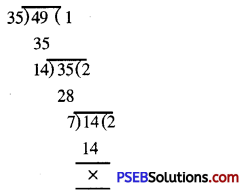So dividing both the numerator and denominator by 7 we get.
$$\frac {35}{49}$$ = $$\frac{35 \div 7}{49 \div 7}$$ = $$\frac {5}{7}$$
∴ Standard form of $$\frac {35}{49}$$ is $$\frac {5}{7}$$

Question (ii).
$$\frac {-42}{56}$$
Solution:
$$\frac {-42}{56}$$
∵ H.C.F. of -42 and 56 is 14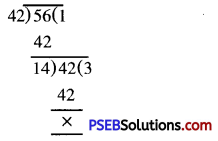So dividing both the numerator and denominator by 14 we get.
$$\frac {-42}{56}$$ = $$\frac{-42 \div 14}{56 \div 14}$$ = $$\frac{-3}{4}$$
∴ Standard form of $$\frac {-42}{56}$$ is $$\frac{-3}{4}$$

Question (iii).
$$\frac {19}{-57}$$
Solution:
$$\frac {19}{-57}$$
∵ H.C.F. of 59 and 57 is 19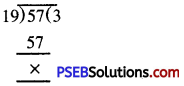So dividing both the numerator and denominator by 19 we get.
$$\frac {19}{-57}$$ = $$\frac{-19 \div 19}{-57 \div 19}$$ = $$\frac{1}{-3}$$
∴ Standard form of $$\frac {19}{-57}$$ is $$\frac{1}{-3}$$Question (iv).
$$\frac{-12}{-36}$$
Solution:
$$\frac{-12}{-36}$$
∵ H.C.F. of 12 and 36 is 12.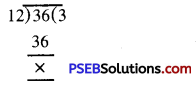So dividing both the numerator and denominator by 12 we get.
$$\frac{-12}{-36}$$ = $$\frac{-12 \div 12}{-36 \div 12}$$ = $$\frac{1}{3}$$
Standard form of $$\frac{-12}{-36}$$ is $$\frac{1}{3}$$

3. Which of the following pairs represent same rational number ?

Question (i).
$$\frac{-15}{25}$$ and $$\frac{18}{-30}$$
Solution:
$$\frac{-15}{25}$$ = $$\frac{-15 \div 5}{25 \div 5}$$
= $$\frac{-3}{5}$$
$$\frac{18}{-30}$$ = $$\frac{18 \div-6}{-30 \div-6}$$
= $$\frac{-3}{5}$$
∴ $$\frac{-15}{25}$$ and $$\frac{18}{-30}$$ represents the same number.

Question (ii).
$$\frac{2}{3}$$ and $$\frac{-4}{6}$$
Solution:
$$\frac{2}{3}$$ = $$\frac{2}{3}$$ × $$\frac{1}{1}$$
= $$\frac{2}{3}$$
$$\frac{-4}{6}$$ = $$\frac{-4 \div 2}{6 \div 2}$$
= $$\frac{-2}{3}$$
∴ $$\frac{-2}{3}$$ and $$\frac{-4}{6}$$ doesnot represents the same rational numbers.

Question (iii).
$$\frac{-3}{4}$$ and $$\frac{-12}{16}$$
Solution:
$$\frac{-3}{4}$$ = $$\frac{-3}{4}$$ × $$\frac{4}{4}$$
= $$\frac{-12}{16}$$
$$\frac{-12}{16}$$ = $$\frac{-12}{16}$$
∴ $$\frac{-3}{4}$$ and $$\frac{-12}{16}$$ represents the same rational number.

Question (iv).
$$\frac{-3}{-7}$$ and $$\frac{3}{7}$$
Solution:
$$\frac{-3}{4}$$ = $$\frac{-3 \div-1}{-7 \div-1}$$
= $$\frac{-3}{4}$$
∴ $$\frac{-3}{-7}$$ and $$\frac{3}{7}$$ represents the same rational number.4. Which is greater in each of the following ?

Question (i).
$$\frac{3}{7}$$, $$\frac{4}{5}$$
Solution:
Given rational nrnnbere are $$\frac{3}{7}$$ and $$\frac{4}{5}$$
L.C.M. of 7 and 5 is 35
∴ $$\frac{3}{7}$$ = $$\frac{3 \times 5}{7 \times 5}$$
= $$\frac{15}{35}$$
and $$\frac{4}{5}$$ = $$\frac{4 \times 7}{5 \times 7}$$
= $$\frac{28}{35}$$
∵ Numerator of second is greater than first i.e. 28 > 15
So $$\frac{4}{5}$$ > $$\frac{3}{7}$$

Question (ii).
$$\frac{-4}{12}$$, $$\frac{-8}{12}$$
Solution:
Given rational numbere are $$\frac{-4}{12}$$ and $$\frac{-8}{12}$$
∵ Numerator of first is greater than second i.e. -4 > – 8
∴ $$\frac{-4}{12}$$ > $$\frac{-8}{12}$$

Question (iii).
$$\frac{-3}{9}$$, $$\frac{4}{-18}$$
Solution:
Given rational numbers are $$\frac{-3}{9}$$, $$\frac{4}{-18}$$
$$\frac{-3}{9}$$ = $$\frac{-3 \times 2}{9 \times 2}$$
= $$\frac{-6}{18}$$
$$\frac{4}{-18}$$ = $$\frac{4 \times-1}{-18 \times-1}$$
$$\frac{-4}{18}$$
Since -4 > – 6.
$$\frac{4}{-18}$$ > $$\frac{-3}{9}$$

Question (iv).
-2$$\frac{3}{5}$$, -3$$\frac{5}{8}$$
Solution:
-2$$\frac{3}{5}$$ = $$\frac{-13}{5} \times \frac{8}{8}$$
= $$\frac{-104}{40}$$
-3$$\frac{5}{8}$$ = $$\frac{-29}{8} \times \frac{5}{5}$$
= $$\frac{-135}{40}$$
∵ -104 > -135
∴ $$\frac{-13}{5}$$ > $$\frac{-29}{8}$$
Thus, -2$$\frac{3}{5}$$ > -3$$\frac{5}{8}$$5. Write the following rational numbers in ascending order.

Question (i).
$$\frac{-5}{7}, \frac{-3}{7}, \frac{-1}{7}$$
Solution:
$$\frac{-5}{7}, \frac{-3}{7}, \frac{-1}{7}$$
Here -5 < -3 < -1
i.e. $$\frac{-5}{7}, \frac{-3}{7}, \frac{-1}{7}$$
Therefore, the ascending order is:
$$\frac{-5}{7}, \frac{-3}{7}, \frac{-1}{7}$$

Question (ii).
$$\frac{-1}{5}, \frac{-2}{15}, \frac{-4}{5}$$
Solution:
$$\frac{-1}{5}, \frac{-2}{15}, \frac{-4}{5}$$
L.C.M of 5, 15, 5 is 15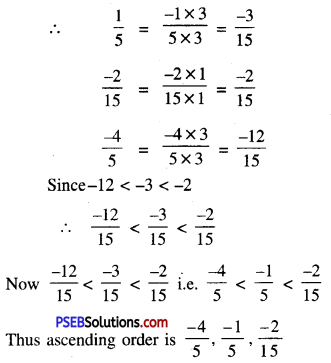Question (iii).
$$\frac{-3}{8}, \frac{-2}{4}, \frac{-3}{2}$$
Solution:
$$\frac{-3}{8}, \frac{-2}{4}, \frac{-3}{2}$$
L.C.M of 8, 4, 2 is 8
∴ $$\frac{-3}{8}=\frac{-3}{8} \times \frac{1}{1}=\frac{-3}{8}$$
$$\frac{-2}{4}=\frac{-2 \times 2}{4 \times 2}=\frac{-4}{8}$$
$$\frac{-3}{2}=\frac{-3 \times 4}{2 \times 4}=\frac{-12}{8}$$
∴ -12 < -4 < -3
or $$\frac {-12}{8}$$ < $$\frac {-4}{8}$$ < $$\frac {-3}{8}$$
Hence assending order is $$\frac{-3}{2}, \frac{-2}{4}, \frac{-3}{8}$$6. Write five rational numbers between following rational numbers.

Question (i).
-2 and -1
Solution:
Given rational numbers are -2 and -1
Let us write -2 and -1 as rational numbers with 5 + 1 = 6 as denominator.
We have -2 = -2 × $$\frac {6}{6}$$
= $$\frac {-6}{6}$$
$$\frac {-12}{6}$$ < $$\frac {-11}{6}$$ < $$\frac {-10}{6}$$ < $$\frac {-9}{6}$$ < $$\frac {-8}{6}$$ < $$\frac {-7}{6}$$ < $$\frac {-6}{6}$$
Hence five rational numbers between -2 and -1 are :
$$\frac {-11}{6}$$,$$\frac {-10}{6}$$,$$\frac {-9}{6}$$,$$\frac {-8}{6}$$,$$\frac {-7}{6}$$
i.e. $$\frac {-11}{6}$$,$$\frac {-5}{3}$$,$$\frac {-3}{2}$$,$$\frac {-4}{3}$$,$$\frac {-7}{6}$$

Question (ii).
$$\frac {-4}{5}$$ and $$\frac {-2}{3}$$
Solution:
Given rational numbers are $$\frac {-4}{5}$$ and $$\frac {-2}{3}$$
First we find equivalent rational numbers having same denominator
Thus $$\frac {-4}{5}$$ = $$\frac{-4 \times 9}{5 \times 9}$$
= $$\frac {-36}{45}$$
and $$\frac {-2}{3}$$ = $$\frac{-2 \times 15}{3 \times 15}$$
= $$\frac {-30}{45}$$
Now, we choose any five integers -35, -34, -33, -32, -31 between the numerators -36 and -30
Then the five rational numbers between $$\frac {-36}{45}$$ and $$\frac {-30}{45}$$ are:
$$\frac{-35}{45}, \frac{-34}{45}, \frac{-33}{45}, \frac{-32}{45}, \frac{-31}{45}$$
Hence, five rational numbers between $$\frac {-4}{5}$$ and $$\frac {-2}{3}$$ are
$$\frac{-35}{45}, \frac{-34}{45}, \frac{-33}{45}, \frac{-32}{45}, \frac{-31}{45}$$
i.e. $$\frac{-7}{9}, \frac{-34}{45}, \frac{-11}{15}, \frac{-32}{45}, \frac{-31}{45}$$

Question (iii).
$$\frac {1}{3}$$ and $$\frac {5}{7}$$
Solution:
Given rational numbers are $$\frac {1}{3}$$ and $$\frac {5}{7}$$
First we find equivalent rational numbers having same denominator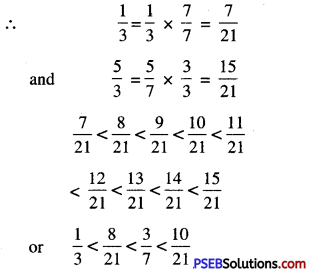$$<\frac{4}{7}<\frac{13}{21}<\frac{2}{3}<\frac{5}{7}$$
Hence, five rational numbers between $$\frac {1}{3}$$ and $$\frac {5}{7}$$ are
$$\frac{8}{21}, \frac{3}{7}, \frac{10}{21}, \frac{4}{7}, \frac{13}{21}$$.7. Write four more rational numbers in each of the following.

Question (i).
$$\frac{-1}{5}, \frac{-2}{10}, \frac{-3}{15}, \frac{-4}{20}, \ldots$$
Solution:
The given rational numbers are :
$$\frac{-1}{5}, \frac{-2}{10}, \frac{-3}{15}, \frac{-4}{20}, \ldots$$
$$\frac {-1}{5}$$ is the rational number in its lowest form
Now, we can write
$$\frac{-2}{10}=\frac{-1}{-5} \times \frac{2}{2}$$,
$$\frac{-3}{15}=\frac{-1}{5} \times \frac{3}{3}$$ and $$\frac{-1}{5}=\frac{-1}{5} \times \frac{4}{4}$$
Thus, we observe a pattern in these numbers.
The next four rational numbers would be
$$\frac{-1}{5} \times \frac{5}{5}=\frac{-5}{25}$$,
$$\frac{-1}{5} \times \frac{6}{6}=\frac{-6}{30}$$,
$$\frac{-1}{5} \times \frac{7}{7}=\frac{-7}{35}$$
$$\frac{-1}{5} \times \frac{8}{8}=\frac{-8}{40}$$
Hence required four more rational numbers are :
$$\frac{-5}{25}, \frac{-6}{30}, \frac{-7}{35}, \frac{-8}{40}$$

Question (ii).
$$\frac{-1}{7}, \frac{2}{-14}, \frac{3}{-21}, \frac{4}{-28}, \ldots$$
Solution:
The given rational numbers are
$$\frac{-1}{7}, \frac{2}{-14}, \frac{3}{-21}, \frac{4}{-28}, \ldots$$
$$\frac {-1}{7}$$ is the rational number in its lowest form
Now, we can write
$$\frac{2}{-14}=\frac{-1}{7} \times \frac{-2}{-2}=\frac{2}{-14}, \frac{3}{-21}$$
= $$\frac{-1}{7} \times \frac{-3}{-3}$$ and $$\frac{4}{-28}=\frac{-1}{7} \times \frac{-4}{-4}$$
Thus, we observe a pattern in these numbers.
The next four rational numbers would be :
$$-\frac{1}{7} \times \frac{-5}{-5}=\frac{5}{-35}$$, $$\frac{-1}{7} \times \frac{-6}{-6}=\frac{6}{-42}$$,
$$\frac{-1}{7} \times \frac{-7}{-7}=\frac{7}{-49}$$, $$\frac{-1}{7} \times \frac{-8}{-8}=\frac{8}{-56}$$
Hence required four more rational numbers are :
$$\frac{5}{-35}, \frac{6}{-42}, \frac{7}{-49}, \frac{8}{-56}$$8. Draw a number line and represent the following rational number on it.

Question (i).
$$\frac {2}{4}$$
Solution:
Draw a line and choose a point O on it to represent the rational number zero (0). We choose a point A to the right of 0 to represent 1.
Divide the segment OA into four equal parts. Second part from O to the right represents the rational number $$\frac {2}{4}$$ as shown in the figure.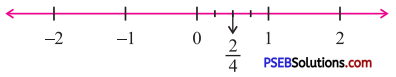Question (ii).
$$\frac {-3}{4}$$
Solution:
Draw a line and choose a point O on it to represent the rational number zero (0). We choose a point A to the right of 0 to represent -1.
Divide the segment OA into four equal parts. Third part from O to the left represents the rational number $$\frac {-3}{4}$$ as shown in the figure.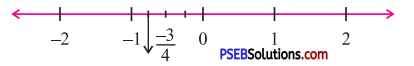Question (iii).
$$\frac {5}{8}$$
Solution:
Draw a line and choose a point O on it to represent the rational number zero (0).
We choose a point A to the right of 0 to represent 1.
Divide the segment OA into eight equal parts. Fifth part from O to the right represents the rational number as shown in the figure.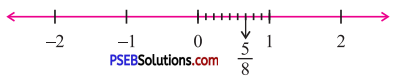Question (iv).
$$\frac {-6}{4}$$
Solution:
Draw a line and choose a point O on it to represent the rational number zero (0).
We choose a point A to the left of O to represent -2.
Divide the segment OA into eight equal parts. Sixth part from O to the left represents the rational number $$\frac {-6}{4}$$ as shown in the figure.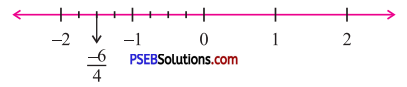9. Multiple Choice Questions :

Question (i).
$$\frac{3}{4}=\frac{?}{12}$$ then ? =
(a) 3
(b) 6
(c) 9
(d) 12.
(c) 9Question (ii).
$$\frac{-4}{7}=\frac{?}{14}$$ then ? =
(a) -4
(b) -8
(c) 4
(d) 8
(b) -8

Question (iii).
The standard form of rational number $$\frac {-21}{28}$$ is
(a) $$\frac {-3}{4}$$
(b) $$\frac {3}{4}$$
(c) $$\frac {3}{7}$$
(d) $$\frac {-3}{7}$$
(a) $$\frac {-3}{4}$$

Question (iv).
Which of the following rational number is not equal to $$\frac {7}{-4}$$ ?
(a) $$\frac {14}{-8}$$
(b) $$\frac {21}{-12}$$
(c) $$\frac {28}{-16}$$
(d) $$\frac {7}{-8}$$
(d) $$\frac {7}{-8}$$

Question (v).
Which of the following is correct ?
(a) 0 > $$\frac {-4}{9}$$
(b) 0 < $$\frac {-4}{9}$$
(c) 0 = $$\frac {4}{9}$$
(d) None
(a) 0 > $$\frac {-4}{9}$$
(a) $$\frac{-4}{5}<\frac{-3}{10}$$
(b) $$\frac{-4}{5}>\frac{3}{-10}$$
(c) $$\frac{-4}{5}=\frac{3}{-10}$$
(a) $$\frac{-4}{5}<\frac{-3}{10}$$